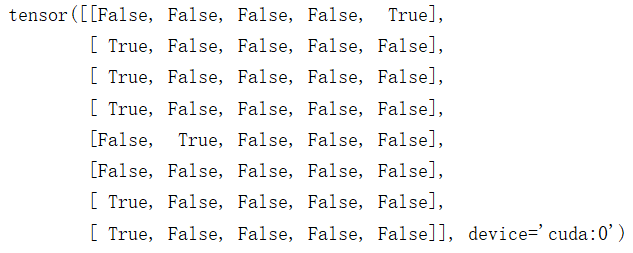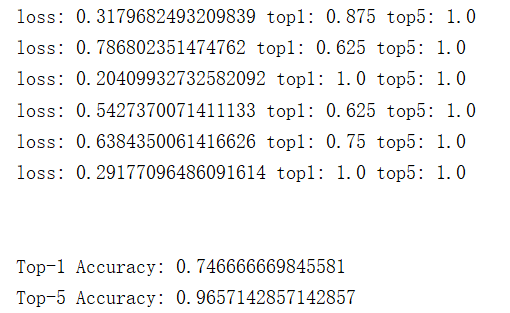# Pytorch 构建 CNN 用于图像分类

### 数据集处理``````class ClsDataset(data_utils.Dataset):
def __init__(self):
pass

def __getitem__(self, item):
pass

def __len__(self):
pass
``````

init方法主要是用于初始化一些类自有的属性，getitem方法主要用于读到数据集中的图片和其对应的标签，并将其转化为可输入CNN的格式，len方法就是返回数据集的大小。``````    def __init__(self,root_path,data_file):
self.root_path=root_path
``````

``````    def __init__(self,root_path,data_file,img_size=256):
self.root_path=root_path
self.class_list=os.listdir(
os.path.join(root_path,'train')
)
``````

``````    def __len__(self):
return len(self.data_files)
``````

``````    def __getitem__(self, item):
data_file=self.data_files[item]
data_file=os.path.join(self.root_path,data_file)
img=Image.open(data_file)
``````

``````		data_file=data_file.replace('/','\\')
tmp=data_file.split('\\')
label_name=tmp[-2]
label=self.class_list.index(label_name)
``````

``````self.transforms=torchvision.transforms.Compose(
[
torchvision.transforms.Resize((img_size,img_size)),
torchvision.transforms.ToTensor()
]
)
``````

``````		img=self.transforms(img)
label=torch.tensor(label)
``````

``````class ClassifyDataset(data_utils.Dataset):
def __init__(self,root_path,data_file,img_size=256):
self.root_path=root_path
self.class_list=os.listdir(
os.path.join(root_path,'train')
)
self.transforms=torchvision.transforms.Compose(
[
torchvision.transforms.Resize((img_size,img_size)),
torchvision.transforms.ToTensor()
]
)

def __getitem__(self, item):
data_file=self.data_files[item]
data_file=os.path.join(self.root_path,data_file)
img=Image.open(data_file)

data_file=data_file.replace('/','\\')
tmp=data_file.split('\\')
label_name=tmp[-2]
label=self.class_list.index(label_name)

img=self.transforms(img)
label=torch.tensor(label)
return img,label

def __len__(self):
return len(self.data_files)
``````

### 网络定义

``````class CNet(nn.Module):
def __init__(self,num_classes=21):
super(CNet,self).__init__()
self.conv1=nn.Sequential(
nn.BatchNorm2d(32),
nn.ReLU()
)
self.conv2 = nn.Sequential(
nn.BatchNorm2d(64),
nn.ReLU()
)
self.conv3 = nn.Sequential(
nn.BatchNorm2d(128),
nn.ReLU()
)
self.conv4 = nn.Sequential(
nn.BatchNorm2d(256),
nn.ReLU()
)
self.conv5 = nn.Sequential(
nn.BatchNorm2d(512),
nn.ReLU()
)
self.pool = nn.AvgPool2d(2, 2)
self.fclayer=nn.Sequential(
nn.Linear(512,1024),
nn.ReLU(),
nn.Linear(1024,num_classes)
)
self.softmax=nn.Softmax(dim=1)
``````

2，且用的是平均池化。

``````    def forward(self,x):
x = self.conv1(x)
x = self.pool(x)
x = self.conv2(x)
x = self.pool(x)
x = self.conv3(x)
x = self.pool(x)
x = self.conv4(x)
x = self.pool(x)
x = self.conv5(x)
x = self.pool(x)
x = self.avg_pool(x)
x = torch.flatten(x,1)
logits=self.fclayer(x)
prob=self.softmax(logits)
return logits,prob
``````

### 训练

``````	root_path = r'自己的路径'
train_data_file= r'自己的路径\train.txt'
test_data_file=r'自己的路径\test.txt'
batch_size=8
lr=0.01
device='cuda:0'
``````

``````device = torch.device("cuda" if torch.cuda.is_available() else "cpu")
``````

``````	model=CNet()
model.to(device)
``````

``````    train_dataset=ClassifyDataset(root_path,train_data_file)
``````

``````	criterion=nn.CrossEntropyLoss()
``````

model.parameters()代表优化全部参数。

``````	epoch_num=10
total_loss=[]
``````

``````        for data in train_dataloader:
train_img,train_label=data
train_img=train_img.to(device)
train_label=train_label.to(device)
``````

``````			train_logits,train_prob=model(train_img)
train_loss=criterion.forward(train_logits,train_label)
``````

``````            optimizer.zero_grad()
train_loss.backward()
optimizer.step()
``````

``````            train_pred=torch.argmax(train_prob,dim=1)
train_acc=(train_pred==train_label).float()
train_acc=torch.mean(train_acc)
print('loss:',train_loss.item(), 'acc:', train_acc.item())
``````

``````    for epoch in range(epoch_num):
## 内层循环代码
if (epoch+1)%5==0:
state_dict=model.state_dict()
torch.save(state_dict,'model.pth')

``````

``````    for epoch in range(epoch_num):
print(epoch+1,"epoch:")
total_train_loss=0
res_num=len(train_dataset)
``````

``````if (res_num - batch_size) > 0:
cnt=batch_size
res_num = res_num - batch_size
else:
cnt=res_num
res_num = 0
``````

``````total_train_loss=total_train_loss+train_loss*cnt
``````

``````total_train_loss=total_train_loss/len(train_dataset)
total_loss.append(total_train_loss.item())
``````

``````	plt.xlabel('epoch')
plt.ylabel('loss')
plt.plot(total_loss)
plt.legend(['train loss'])
plt.show()
````````````# 训练代码
train_dataset=ClassifyDataset(root_path,train_data_file)

criterion=nn.CrossEntropyLoss()

epoch_num=20
total_loss=[]

for epoch in range(epoch_num):
print(epoch+1,"epoch:")
total_train_loss=0
res_num=len(train_dataset)
if (res_num - batch_size) > 0:
cnt=batch_size
res_num = res_num - batch_size
else:
cnt=res_num
res_num = 0

train_img,train_label=data
train_img=train_img.to(device)
train_label=train_label.to(device)

train_logits,train_prob=model(train_img)
train_loss=criterion.forward(train_logits,train_label)

train_loss.backward()
optimizer.step()

train_pred=torch.argmax(train_prob,dim=1)
train_acc=(train_pred==train_label).float()
train_acc=torch.mean(train_acc)
print('loss:',train_loss.item(), 'acc:', train_acc.item())
total_train_loss=total_train_loss+train_loss*cnt

total_train_loss=total_train_loss/len(train_dataset)
total_loss.append(total_train_loss.item())

# if (epoch+1)%5==0:
#     state_dict=model.state_dict()
#     torch.save(state_dict,'model_100_epoch.pth')

plt.xlabel('epoch')
plt.ylabel('loss')
plt.plot(total_loss)
plt.legend(['train loss'])
plt.show()
``````

### 测试

``````# 测试代码
model.eval()

test_dataset=ClassifyDataset(root_path,test_data_file)

criterion = nn.CrossEntropyLoss()

res_num=len(test_dataset)
total_acc=0
total_top5_acc=0

if (res_num-batch_size)>0:
cnt=batch_size
res_num = res_num - batch_size
else:
cnt=res_num
res_num = 0

test_img,test_label=data
test_img=test_img.to(device)
test_label=test_label.to(device)

test_logits,test_prob=model(test_img)
test_loss=criterion.forward(test_logits,test_label)

# Top1 准确率
test_pred = torch.argmax(test_prob, dim=1)
test_acc = (test_pred == test_label).float()
test_acc = torch.mean(test_acc)
total_acc = total_acc + test_acc * cnt

# Top5 准确率
_,test_top5_pred=test_logits.topk(5,1,True,True)
test_top5_acc=torch.eq(test_top5_pred,test_label.view(-1, 1)).sum().float().item()
total_top5_acc=total_top5_acc+test_top5_acc

print('loss:',test_loss.item(), 'top1:',test_acc.item() ,'top5:',test_top5_acc/cnt)

total_acc=total_acc/len(test_dataset)
total_top5_acc=total_top5_acc/len(test_dataset)
print('\n')
print('Top-1 Accuracy:',total_acc.item())
print('Top-5 Accuracy:', total_top5_acc)
``````

top5准确率就相对来说比较复杂，我们这里借助了pytorch的自带的函数topk。官方中文文档给出的介绍如下：``````_,test_top5_pred=test_logits.topk(5,1,True,True)
``````

``````print(torch.eq(test_top5_pred,test_label.view(-1, 1)))
``````